C Program to Add Two Numbers using Pointer

In this tutorial you will learn about the C Program to Add Two Numbers using Pointer and its application with practical example.

In this tutorial, we will learn to create a C program that  will add two number using pointer in C programming.

Prerequisites

Before starting with this tutorial we assume that you are best aware of the following C programming topics:

• C Operators.
• Basic input/output.
• Pointers.
• Basic C programming.

What Is Pointer?

A pointer variable are who hold the  address of another variable.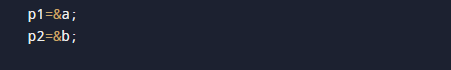C Program to Add Two Numbers using Pointer.

In this program first of all we take values from user and passes the address or value to the pointer variable and without using the actual variable we will calculate addition of them using pointer .let see the code now.

Output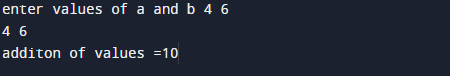Explanation.

In the program, we have  2 integer variables a and b and 2 pointer variables *p1 and *p2 . Now we assign the addresses of a and b to p1 and p2 receptively.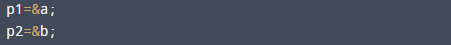and then adding value of a and b using *p1 and *p2 into  add.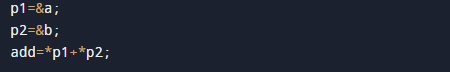Herevariable p1 holds the address of variable a with &(address) operator and p2 holds the address of variable b. So here & operator provide the address of variables.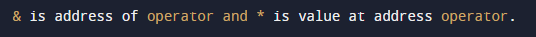and with the help of *(Asterisk) operator we can access the value of given address using pointer variable.as we can see in above image p1 holds the address of variable a and p2 holds the address of variable b and without using a and b we are adding the values of a and b  using pointer.

So in this program we have calculate two values using pointer variables .

In this tutorial we have learn about the C Program to Add Two Numbers using Pointer and its application with practical example. I hope you will like this tutorial.